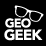# Creating Field Calculator Expression

4216
6
01-13-2015 01:06 AMNew Contributor

Hi, I want to project population from an old census report.....the formula is as follows

population*(1+(growth rate/100))^16

I am trying this expression for calculating from attribute table but ITS NOT WORKING

[POP_B_SEX]*(1+ (4.33 / 100 ))Exp (16 )

Note : [POP_B_SEX] is the only column i am using from attribute table.

1 Solution

Accepted SolutionsbyEsri Contributor

The formula works for me....

Go in ArcMap to the Geoprocessing menu and click Geoprocessing Results. What is the error message that the Field Calculator returns?

To make the formula more simple:

(1+ (4.33 / 100 ))^16 = 1.97037

Your formula can be rewritten as:

[POP_B_SEX]*1.97037

6 RepliesbyMVP Legendary Contributor

Exponents aren't ^, they are **New Contributor

Yes but you see in the expression i am using "exp()"...wont they work?? and i tried with ** and doesn't work either....i guess there is something wrong with the expression....byEsri Contributor

Exp(16) means e^16 = 2.718282^16 = 8886111

You can simply use the ^ sign as in the formula.

Note: there is a difference between:

[POP_B_SEX]*(1+ (4.33 / 100 ))^16

and

([POP_B_SEX]*(1+ (4.33 / 100 )))^16New Contributor

Yeah i tried with ^ already but it doesn't workbyEsri Contributor

The formula works for me....

Go in ArcMap to the Geoprocessing menu and click Geoprocessing Results. What is the error message that the Field Calculator returns?

To make the formula more simple:

(1+ (4.33 / 100 ))^16 = 1.97037

Your formula can be rewritten as:

[POP_B_SEX]*1.97037New Contributor

Yes it worked i had an extra square bracket..thanks